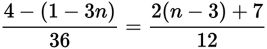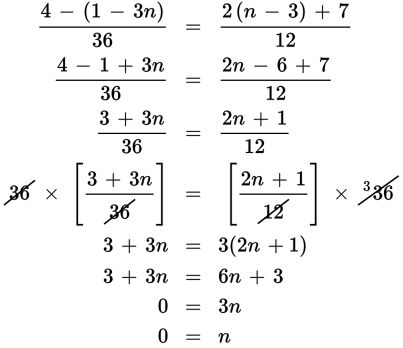# SAT Math Multiple Choice Question 278: Answer and Explanation

### Test Information

Question: 278

8.In the equation above, what is the value of n?

• A. 0
• B. 2
• C. 3
• D. There is no value of n that satisfies the equation.

Explanation:

A

Difficulty: Medium

Category: Heart of Algebra / Linear Equations

Strategic Advice: Choose the best strategy to answer the question-cross-multiplying in this question (without a calculator) will create large numbers and possibly lead to errors. Instead, start by simplifying the numerators and then multiply both sides of the equation by the least common denominator, 36.